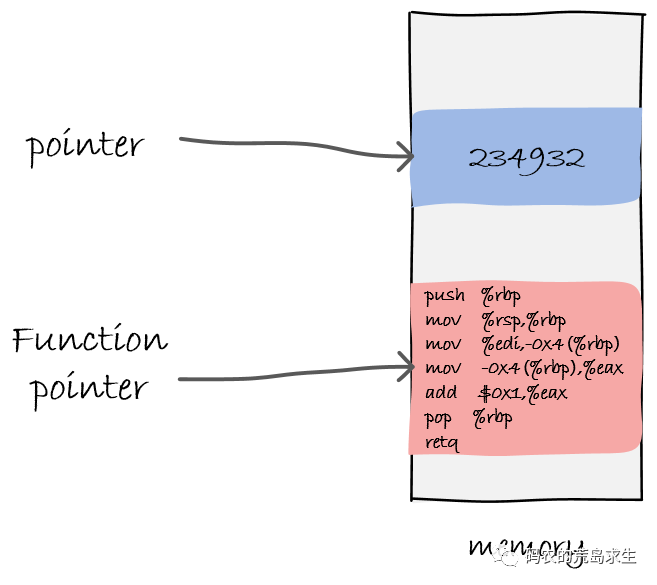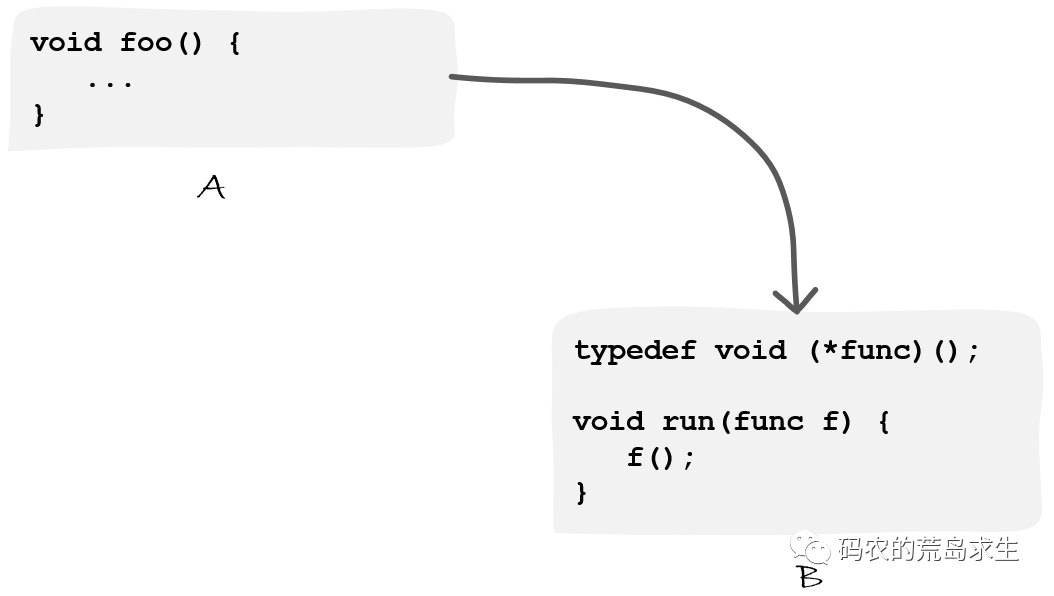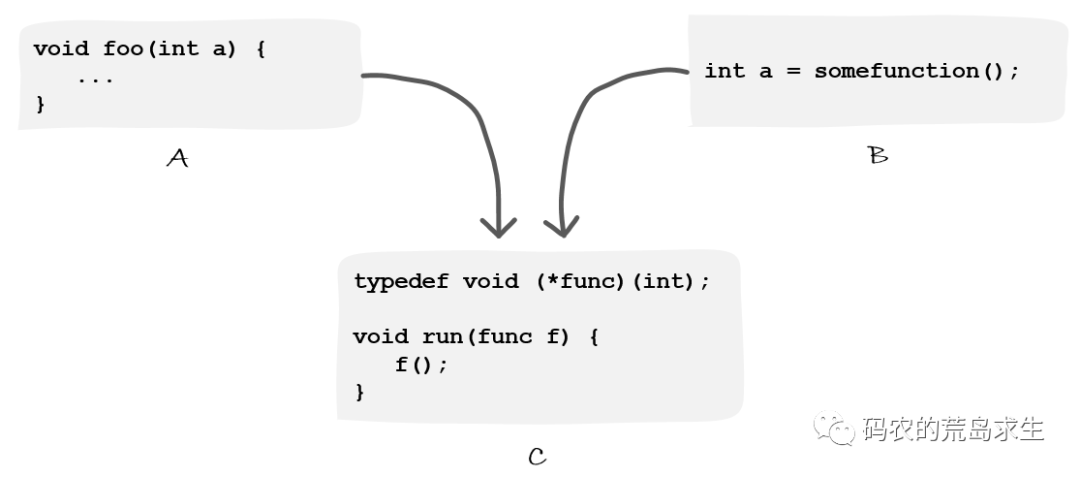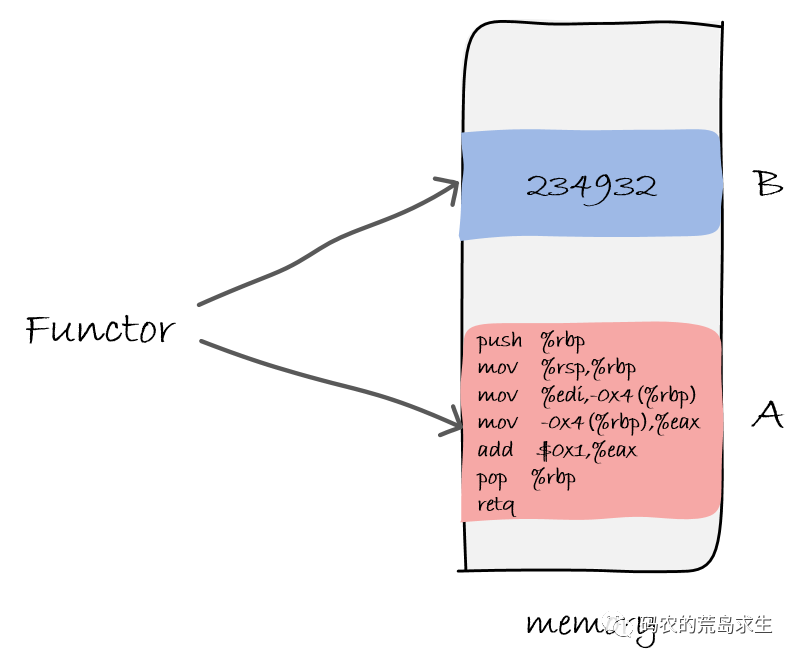In C/C++ you can use a pointer to a piece of code, which is called a function pointer, suppose there is a piece of code like this.

 `````` 1 2 3 4 5 6 7 8 9 10 `````` ``````#include int func(int a) { return a + 1; } void main() { int (*f)(int) = func; printf("%p\n", f); } ``````

We define a function func, then use the pointer variable f to point to the function, then print out the address pointed to by the variable f. The code is simple, then we compile it and look at the instructions generated after compilation, we focus on the func function.

 ``````1 2 3 4 5 6 7 8 `````` ``````0000000000400526 : 400526: 55 push %rbp 400527: 48 89 e5 mov %rsp,%rbp 40052a: 89 7d fc mov %edi,-0x4(%rbp) 40052d: 8b 45 fc mov -0x4(%rbp),%eax 400530: 83 c0 01 add \$0x1,%eax 400533: 5d pop %rbp 400534: c3 retq ``````

As you can see, the compiled function func is located at the address 0x400526, so let’s remember that address.

Then run the compiled program and think about what this code will output.

Obviously it should be the address of the func function in memory!

 ``````1 2 `````` ``````\$ ./a.out 0x400526 ``````

You guessed it right, but a function pointer is essentially a pointer, except that it points to a piece of code in memory instead of a piece of data in memory, like this:See, what we often call a pointer generally points to a piece of data in memory, while a function pointer points to a piece of code in memory, in this example pointing to memory address 0x400526, where the machine instruction for function func is stored.

Now that you should understand the function pointer, a careful person may have a question, Why does the compiler know that the function func is stored at memory address 0x400526 when it generates the executable file? Shouldn’t this be determined only when the program is loaded into memory and starts running?

The function pointer is used to pass a piece of code around as a variable, and one of its main uses is to call back functions.

Regarding callback functions that are actually defined in module A and called in module B, like this.However, sometimes we have a scenario where we still need to define a function in module A, while the operation of function A needs to rely on the data generated by module B. Then the function defined in module A and the data generated by module B are passed together to module C to be called, like this.At this point, a simple function pointer is no longer enough, because the function pointer simply points to a piece of code in memory, we not only need to pass a piece of code in memory but also a piece of data in memory to module C. At this point you can define a structure to package the code and data , like this.

 ``````1 2 3 4 5 6 `````` ``````typedef void (*func) (int); struct closure{ func f; int arg; }; ``````

Let’s name this structure closure and notice that there are two parts in this structure.

• a pointer variable to the code
• a variable that holds the data

Thus, we assign a value to the pointer variable in module A and a value to the variable that holds the data in module B. We then pass this structure to module C, where it can be used as follows.

 ``````1 2 3 `````` ``````void run(struct functor func) { func->f(func->arg); } ``````

That is, closure contains both a piece of code and the data used by this code, which is also called context or environment, whatever it is called, but is actually the data on which the function runs.And that’s exactly what std::function is for in C++.

Mere function pointers don’t have the ability to capture context, where context means code-dependent data, and you have to construct your own structure to store the code-dependent context.

The reason you don’t have a way in C++ to simply use function pointers to point to member functions of an object is that there is no way for function pointers to capture the context of this (a pointer to an object).

What std::function does is essentially not much different from the structure we just defined.

With std::function you can not only store a piece of code, but also the necessary context, and then call the code based on that context in the right place.

Also std::function is more general, you can use it to store any callable object, as long as it has the correct function signature.